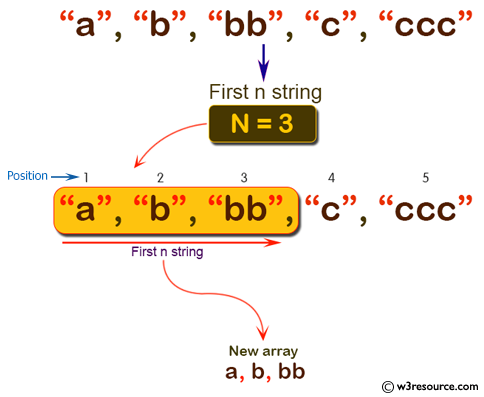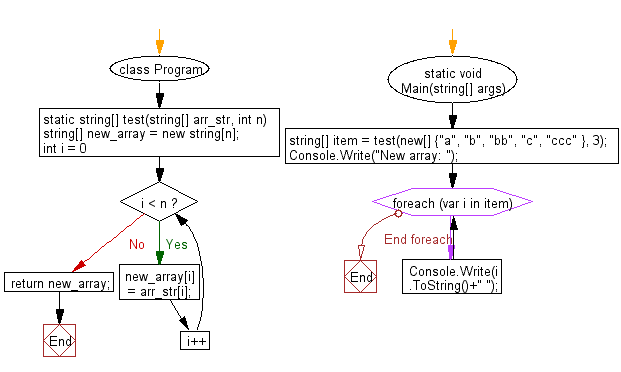﻿ C# - Create an array using the first n strings from an array# C# Sharp Basic Algorithm Exercises: Create a new array using the first n strings from a given array of strings

## C# Sharp Basic Algorithm: Exercise-137 with Solution

Write a C# Sharp program to create a new array using the first n strings from a given array of strings. (n>=1 and <=length of the array)

Pictorial Presentation:Sample Solution:

C# Sharp Code:

``````using System;
using System.Collections;
using System.Collections.Generic;
using System.Linq;
using System.Text;
namespace exercises
{
class Program
{
static void Main(string[] args)
{

string[] item = test(new[] {"a", "b", "bb", "c", "ccc" }, 3);
Console.Write("New array: ");
foreach(var i in item)
{
Console.Write(i.ToString()+" ");
}

}

static string[] test(string[] arr_str, int n)
{
string[] new_array = new string[n];

for (int i = 0; i < n; i++)
{
new_array[i] = arr_str[i];
}

return new_array;
}

}
}
```
```

Sample Output:

```New array: a b bb
```

Flowchart:C# Sharp Code Editor:

Improve this sample solution and post your code through Disqus

What is the difficulty level of this exercise?

Test your Programming skills with w3resource's quiz.

﻿